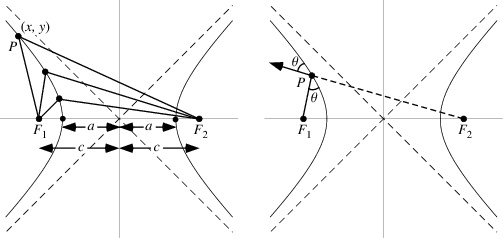# Cartesian form of a Hyperbola

## Homework Statement

Why is it necessarily true that for a hyperbola, the focus length, ##f ## has got to be greater than the semi-major axis , ## a## - ## f >a ## ?

-

## The Attempt at a Solution

I needed to derive the cartesian equation of a hyperbola with centre at ## (\alpha,\beta) ## and foci along the ##\alpha - ## axis. using the definition that the difference between the distances to the two foci is a constant. After some algebra, I just got back the equation of an ellipse.

$$\frac{(x-\alpha)^{2}}{a^{2}}+\frac{(y-\beta)^{2}}{a^{2}-f^{2}}=1$$

Of course I realise that this is also the equation of a hyperbola, under the condition that ## f>a ## so that :
$$\frac{(x-\alpha)^{2}}{a^{2}}-\frac{(y-\beta)^{2}}{a^{2}-f^{2}}=1$$

But I'm stuck at proving that this condition is, in general true, just from the definition given.

Could someone give me a hint?

Thanks!

mfb
Mentor
I think you forgot to swap the signs in the denominator in the second equation.

We just call shapes with f>a "hyperbola" and with f<a "ellipse", they get different names because they look so different. In particular, a hyperbola has solutions for arbitrarily large x and y, which means the two summands must have different signs, whereas they have the same sign for an ellipse.

Yes, sorry I did! So the definition of the hyperbola as being the locus where there is a constant difference between the distances to two foci is incomplete? I need to know that ## f>a ## on top - I can't prove that just from this definition?

mfb
Mentor
f>a should follow from that requirement.

That's my difficulty, I don't know how to show that it must follow... :P

In full my working currently looks like this - I'll borrow this convenient wolfram diagram for the symbols. Although the diagrams show clearly that ## f>a ## I do not think I assume this in my calculation. I've dropped the shift in the centre of the co-ordinate system because it doesn't really matter and obscures the working. (so centre is now (0,0) not ##(\alpha, \beta ) ##Let ## r_{2}= F_{2}P \ , \ r_{1} = F_{1}P ##

$$r_{2}-r_{1} =C \implies \sqrt{(x-f)^{2}+y^{2}}-\sqrt{(x+f)^{2}+y^{2}} =C$$
$$\therefore (x-f)^{2}+y^{2} = C^{2}+(x+f)^{2}+y^{2}+2C\sqrt{(x+f)^{2}+y^{2}}$$
$$\implies -4xf=C^{2}+2C \sqrt{(x+f)^{2}+y^{2}}$$
$$(C+4x \frac{f}{C})^{2}=4(x+f)^{2}+4y^{2}$$
$$C^{2}+16\frac{x^{2}f^{2}}{C^{2}}+8xf = 4x^{2}+8xf+4f^{2} + 4y^{2}$$
$$C^{2}-4f^{2} = 4x^{2}(\frac{C^{2}-4f^{2}}{C^{2}})+4y^{2} \implies \frac{4x^{2}}{C^{2}}+\frac{4y^{2}}{C^{2}-4f^{2}} =1$$

From above ,at ## y =0 \ , \ 2x =C ## By definition of the semi-major axis of the hyperbola, if ## y= 0 \implies x=a ##

$$\therefore \frac{x^{2}}{a^{2}}+\frac{y^{2}}{a^{2}-f^{2}}=1$$

But from here I can't see how to retrieve the minus sign.
Thanks!!

mfb
Mentor
If C>2f (which corresponds to f<a as 2a=C), you do not get any solution of your original equation. The squaring process fakes an additional solution which has a meaning for an ellipse, but not here.